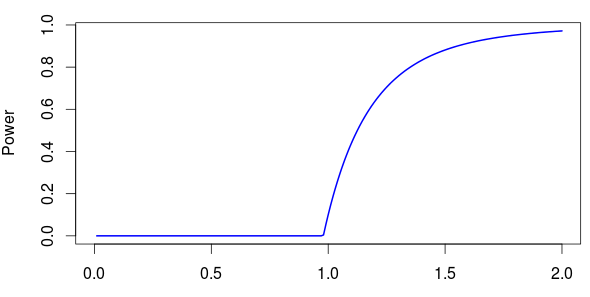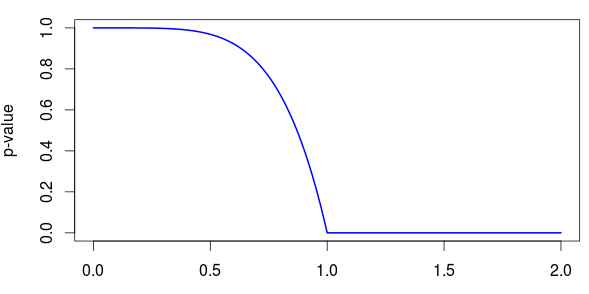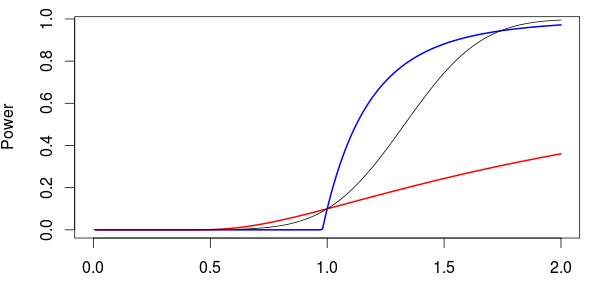Want to share your content on R-bloggers? click here if you have a blog, or here if you don't.

In the mathematical statistics course today, we started talking about tests, and decision rules. To illustrate all the concepts introduced today, we considered the case where we have a sample  with . And we want to test

against

In the course, we’ve seen that we could use a test based on the order statistics .  The test would be

i.e. if  we choose , and if , we choose .

From the definition of the first order risk,

we can easily get that

Thus, the power is then

To visualize it, use the following parameters

```n=5
alpha=.1
theta0=1```

Then

```C1=theta0*(1-alpha)^(1/n)
theta=seq(0,2,by=.01)
P1=(1-(theta0/theta)^n*(1-alpha))*(theta>C1)
plot(theta,P1,type="l",lwd=2,col="blue",xlab="",ylab="Power")```Note that, so far, we did never consider the maximum of our sample. Assume that the maximum is , then we can compute the -value,

Here it is

```PV=(1-theta^n)*(theta<=1)
plot(theta,PV,type="l",lwd=2,col="blue",xlab="",ylab="p-value")```Now, why not consider another test, based on the minimum (since we have the distribution of the minimum of a sample from a uniform distribution). The test is the same as before

but here, the threshold is

The power of the test is here

This test has the same significance level (by construction), but the power of the test is clearly lower than the one we got using the maximum of our sample, when

```C2=theta0*(1-alpha^(1/n))
P2=(1-(theta0/theta)*(1-alpha^(1/n)))^n*(theta>C2)
lines(theta,P2,type="l",lwd=2,col="red")```Why not consider a test based on ? The problem is that we need the distribution (more specifically the survival distribution) of . We can compute it, numerically. But that might be painful. An alternative is to consider some approximation, based on the central limit theorem, i.e.

Our test is based on , and to get the same significance as before, use

The power of the test is then

Here it is

```mu=2*(theta0/2)
s2=2^2*(theta0^2/12)/n
C3=qnorm(1-alpha,mu,sqrt(s2))
(P=1-pnorm(C3,theta,sqrt(s2)))*(theta>C3)
lines(theta,P)```Observe here that the test based on the maximum is not more powerful than the one based on the average (I just wonder if it could be due to the Gaussian approximation…).